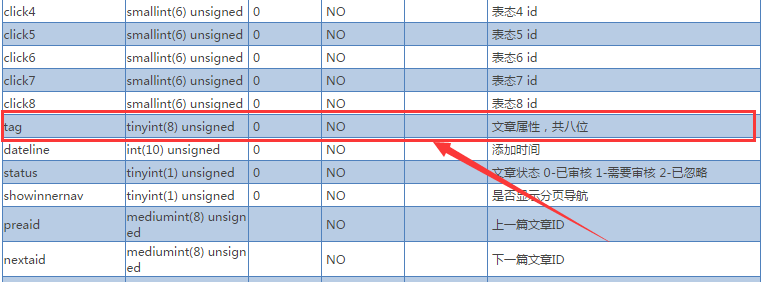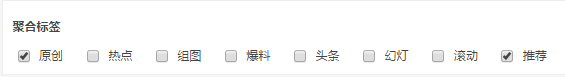# Discuz门户文章8个标签属性的数据库存储分析

2015-11-29 15:03 来自：Discuz 评论(0) 查看(2,074)

Discuz不用多说，大家应该很多人都了解，国内知名社区论坛程序，为腾讯旗下康盛公司开发（被腾讯收购的），已经有十多年的发展，确实是一款优秀的产品。虽然它的主要卖点是论坛功能，但同时还有sns功能、门户功能。```function article_parse_tags(\$tag) {
\$tag = intval(\$tag);
\$article_tags = array();
for(\$i=1; \$i<=8; \$i++) {
\$k = pow(2, \$i-1);
\$article_tags[\$i] = (\$tag & \$k) ? 1 : 0;
}
return \$article_tags;
}

function article_make_tag(\$tags) {
\$tags = (array)\$tags;
\$tag = 0;
for(\$i=1; \$i<=8; \$i++) {
if(!empty(\$tags[\$i])) {
\$tag += pow(2, \$i-1);
}
}
return \$tag;
}
```

```if(is_array(\$tag)) {
\$article_tags = array();
foreach(\$tag as \$k) {
\$article_tags[\$k] = 1;
}
include_once libfile('function/portalcp');
\$v=article_make_tag(\$article_tags);
if(\$v > 0) {
\$wheres[] = "(at.tag & \$v) = \$v";
}
}
```

============================华丽的分割线============================`\$tags=array(0=>1,1=>0,2=>0,3=>0,4=>0,5=>0,6=>0,7=>1);`

`\$article_tags=array(0=>1,1=>0,2=>0,3=>0,4=>0,5=>0,6=>0,7=>1);`

`\$tag=array(0=>1,1=>0,2=>0,3=>0,4=>0,5=>0,6=>0,7=>0);`